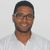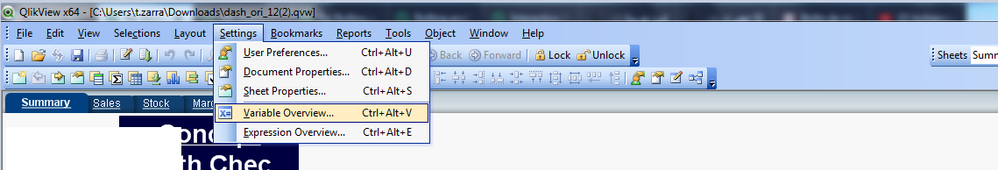# QlikView App Dev

Discussion Board for collaboration related to QlikView App Development.

Announcements
Now Live: Qlik Sense SaaS Simplified Authoring – Analytics Creation for Everyone: READ DETAILS
cancel
Showing results for
Did you mean:Contributor III

## Rolling and YTD calculation

Hello,

I'm trying to calculate the rolling and ytd revenue, but not from the month of january to december. i need to do it from September 2017 to aug 2018 and sep 2018 to aug 2019. i'm using below code to ge the output. I have a filter called view in which i have two selection rolling and ytd. if i select rolling then the in the revenue text object the output should be current year rolling and last year revenue, if i select ytd from the view filter, then the revenue text object should de displayed as current year YTD and last year ytd revenue. with the below i'm not getting the desired output, the numbers are not correct.

='Current Year:'& Num(Sum({<[Trading Week End Date] = {">=\$(=MakeDate(Year(Today()),9,1) )<=\$(=MakeDate(Year(Today())+1,8,1) )"}> }>} NET_SLS_AMT/1000000),'##.00')& ' Last Year:'&Num(Sum({<[Trading Week End Date] = {">=\$(=MakeDate(Year(Today())-1,9,1) )<=\$(=MakeDate(Year(Today()),8,1) )"}> } NET_SLS_AMT/1000000),'##.00')

18 RepliesMVP

49.11 for last year ?

Regards,
Taoufiq ZARRA

"Please LIKE posts and "Accept as Solution" if the provided solution is helpful "

(you can mark up to 3 "solutions") 😉Contributor III
Author

yes, correct. 49.11 for the last yearMVP

Regards,
Taoufiq ZARRA

"Please LIKE posts and "Accept as Solution" if the provided solution is helpful "

(you can mark up to 3 "solutions") 😉MVP

d1=Date(Makedate(Year(Max(week))-1,09,01),'DD/MM/YYYY')

d2=Date(Makedate(Year(Max(week)),08,01),'DD/MM/YYYY')

d3=Date(Makedate(Year(Max(week))-2,09,01),'DD/MM/YYYY')

d4=Date(Makedate(Year(Max(week))-1,08,01),'DD/MM/YYYY')

then change revenue text by

``````='Current Year:'& Num((Sum({<week = {">=\$(d1) <=\$(d2)"}>} NET_SLS_AMT)/1000000),'##.00')
& ' Last Year:'&num((((Sum({<week = {">=\$(d3)<=\$(d4)"},Period=>} NET_SLS_AMT)/1000000)
-
Sum({<week = {">=\$(d3)<=\$(d4)"},Period= {'LYTD'}> } NET_SLS_AMT)/1000000)),'##.00')``````

Regards,
Taoufiq ZARRA

"Please LIKE posts and "Accept as Solution" if the provided solution is helpful "

(you can mark up to 3 "solutions") 😉Contributor III
Author

Hi

where should i create these variables?  a text object

d1=Date(Makedate(Year(Max(week))-1,09,01),'DD/MM/YYYY')

d2=Date(Makedate(Year(Max(week)),08,01),'DD/MM/YYYY')

d3=Date(Makedate(Year(Max(week))-2,09,01),'DD/MM/YYYY')

d4=Date(Makedate(Year(Max(week))-1,08,01),'DD/MM/YYYY')MVPthenRegards,
Taoufiq ZARRA

"Please LIKE posts and "Accept as Solution" if the provided solution is helpful "

(you can mark up to 3 "solutions") 😉Contributor III
Author

Hi,

swap1546@gmail.com is my mail id.

the number for rolling is coming correct, but when i select YTD from view filter the numbers are not correct. attaching you the screenshot.MVP

what are the correct values?

Regards,
Taoufiq ZARRA

"Please LIKE posts and "Accept as Solution" if the provided solution is helpful "

(you can mark up to 3 "solutions") 😉Contributor III
Author

Hi,

Thanks a ton for guiding me to get the desired output. i just have a small problem here. With the below and making variable overview. my YTD last year revenue is coming upto be zero. Can you please through a light on this.

Attaching you the screenshot of the same.='Current Year:'& Num((Sum({<week = {">=\$(d1) <=\$(d2)"}>} NET_SLS_AMT)/1000000),'##.00')
& ' Last Year:'&num((((Sum({<week = {">=\$(d3)<=\$(d4)"},Period=>} NET_SLS_AMT)/1000000)
-
Sum({<week = {">=\$(d3)<=\$(d4)"},Period= {'LYTD'}> } NET_SLS_AMT)/1000000)),'##.00')

d1=Date(Makedate(Year(Max(week))-1,09,01),'DD/MM/YYYY')

d2=Date(Makedate(Year(Max(week)),08,30),'DD/MM/YYYY')

d3=Date(Makedate(Year(Max(week))-2,09,01),'DD/MM/YYYY')

d4=Date(Makedate(Year(Max(week))-1,08,30),'DD/MM/YYYY')

This will be big help for me.

Thanks

Sai